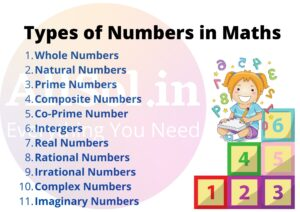# Types of Numbers in Maths### Whole Numbers:-

The natural numbers along with zero form the collection of whole numbers. In other words Numbers Starting from 0 are called Whole numbers.

The whole number set is represented by the letter “W”.

### Natural Numbers:-

The numbers 1, 2, 3,4,5,… which we use for counting are known as natural numbers. In other words Numbers Starting from 1 are called Natural numbers.

The Natural Number set is represented by the symbol “N”.

Point to be noted:-  All natural numbers are whole numbers, but all whole numbers are not natural

Numbers as 0 does not comes in the category of Natural Numbers.

## Even Numbers:-

### Prime Numbers:-

The numbers other than 1 whose only factors are 1 and the number itself are called Prime numbers.

2 is the smallest prime number which is even.

Every prime number except 2 is odd.

### Composite Numbers:-

Numbers having factors other than one and itself are called Composite Numbers. Numbers having more than two factors are called Composite numbers.

Point to be noted : – 1 is neither a prime nor a composite number.

### Co-Prime Number:-

Two numbers with only 1 as a common factor are called co-prime numbers.

### Intergers:-

The set of all whole numbers with a negative set of natural numbers is known as Integers. The integer set is represented by the symbol “Z”.

### Real Numbers:-

Any number whether it is positive integers, negative integers, fractional numbers or decimal numbers excluded imaginary numbers are called the real numbers. It is represented by the letter “R”.

### Rational Numbers:-

Any number that can be written in the form of p/q, where q ≠0, means a ratio of one number over another is known as rational numbers. A rational number can be represented by the letter “Q”.

### Irrational Numbers:-

The number that cannot be expressed in the form of p/q is called Irrational number. It is represented by the letter ”P”.

It means a number that cannot be written as the ratio of one number over another number is known as irrational numbers.

### Complex Numbers:-

A number that is in the form of a+bi is called complex numbers, where “a and b” should be a real number and “i”should be an imaginary number.

### Imaginary Numbers:-

The imaginary numbers comes under complex numbers. It is the product of real numbers with an imaginary unit “i”. The imaginary part of the complex numbers is defined by Im (Z).